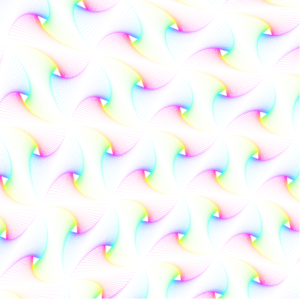# WebGL Zen DoodleWebGL Zen Doodle Screenshot. Click link below for live demo.

This new WebGL demo demonstrates the drawing of a triangular zen doodle. Zen doodles are usually small drawings of arbitrary patterns drawn to relax or think.

The demo uses a simple triangle pattern which is created by starting with a triangle. Then, for a certain amount of times, a new triangle is created. Each new triangle (child) is rotated and then scaled to fit within the previously generated triangle (parent). There are other ways to create this pattern but this one very simple. To make things pretty, colors get more saturated to the inside and are animated. The vertices move and change size using some sine waves and the amount of rotation is also animated, giving the image a slow breathing feeling.

From the technical side, figuring out the scaling amount after each rotation is a bit tricky. To make it a bit easier I simply used triangles where all sides have the same length, which allows me to use a uniform scale. To calculate the scaling amount, the intersection of the direction vector (=line from the origin to the rotated  first vertex of the parent triangle) with the right side (assuming clockwise rotation) of the parent triangle is calculated. Since everything is calculated relative to the origin, the new scale is given by the length of the intersection vector.

WebGL Zen Doodle